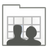### Enumerating Active Directory Group Members with PerlSomeone just asked me for help reading the members of a group. Pretty basic function after all, and you know, it's not as simple as you might think.  That's not to say that it takes much code, but there's a bit of confusion about how it's done.  The confusion comes from the fact that there are two ways to get the members of a group.  The member attribute, and the members method.

If you look at a group object with adsiedit, you'll find that there is an attribute called member.  This attribute contains a list of strings, which are the distinguished names of the members of the group.  The key word here is list.  When you get the member attribute in your script, you get a reference (a pointer) to an array.  That is, you get the memory address of an array.  For example, let's try this code, and see the results.

```use Win32::OLE('in');
```
```\$group=Win32::OLE->GetObject("LDAP://cn=domain admins,cn=users,dc=myDomain,dc=com");
```
```print "\$group->{member}\n";
```

When you run this, you get the following output (the memory address will vary of course):

ARRAY(0x1991ecc)

Not very useful.  However, if you cast that array reference to an array, using @{} the array contents become accessible to you, and you can then access them using foreach. Like so:

```use Win32::OLE('in');
```
```\$group=Win32::OLE->GetObject("LDAP://cn=domain admins,cn=users,dc=myDomain,dc=com");
```
```foreach \$member (@{\$group->{member}}){
print "\$member\n";
}```

As you can see (although it looks a little ugly with all those curly braces), I casted \$group->{member} to an array by wrapping it in @{}, resulting in @{\$group->{member}}, which gave us a list of the members' DNs.

Now that's great, if you really want the DNs.  DNs are useful if you intend to use the DNs to go get each member and do something with them, but if you want to simply print out the members' name, then you have to call getObject on each member to get their name.  That'll take more code and more time.  We're better of doing things another way, using the members method.

Let's try another piece of code, this time using the members method rather than the member attribute:

```use Win32::OLE('in');
```
```\$group=Win32::OLE->GetObject("LDAP://cn=domain admins,cn=users,dc=americas,dc=astrazeneca,dc=net");
```
`print "\$group->{members}\n";`

Now we see a different (but equally useless) result:

Win32::OLE=HASH(0x1991eec)

This is a reference to an object, or rather an object collection.  In other words, it's a list of the user objects that are members of the group.  To access the items in this collection, we enumerate the collection.  In this case, foreach needs a little help.  Perl needs to get the enumerator interface for the object collection.  We do this using the simple in symbol.  Notice that we imported the in symbol in the first line of the script.

When we walk through the collection with foreach, each item is a user object, and we can simply access the user's attributes on the fly.

```use Win32::OLE('in');
```
```\$group=Win32::OLE->GetObject("LDAP://cn=domain admins,cn=users,dc=myDomain,dc=com");
```
```foreach \$member (in \$group->{members}){
print "\$member->{cn}\n";
}```

Ah, now I bet that's what you were looking for.  You can easily change the print line to display a different attribute, such as display name, like this print "\$member->{displayName}\n";

This method is much faster than using the member attribute, getting each user object, and retrieving the user's display name, and this is the method I almost always use.